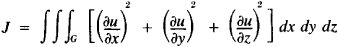# Dirichlet Principle

(redirected from Dirichlet's principle)
Also found in: Wikipedia.

## Dirichlet Principle

(named after P. G. L. Dirichlet). (1) The box principle—the proposition that states that if m > n for each of m objects assigned to one of n classes, at least two objects will fall into at least one class. This extremely simple proposition is used to prove many important theorems in the theory of numbers pertaining to the approximation of irrational numbers by rational numbers, to prove the transcendence of numbers, and so forth.

(2) In the theory of harmonic functions the following propositions is called the Dirichlet principle: among all possible functions assuming given values on the boundary of region G, the function for which the integralattains the least value will be harmonic on the interior of G. This proposition has a simple physical meaning (if u is the potential of velocities in a steady flow of a homogeneous incompressible fluid, then J expresses with an accuracy to a constant factor the kinetic energy of the fluid). The Dirichlet principle is widely used in mathematical physics.

Site: Follow: Share:
Open / Close